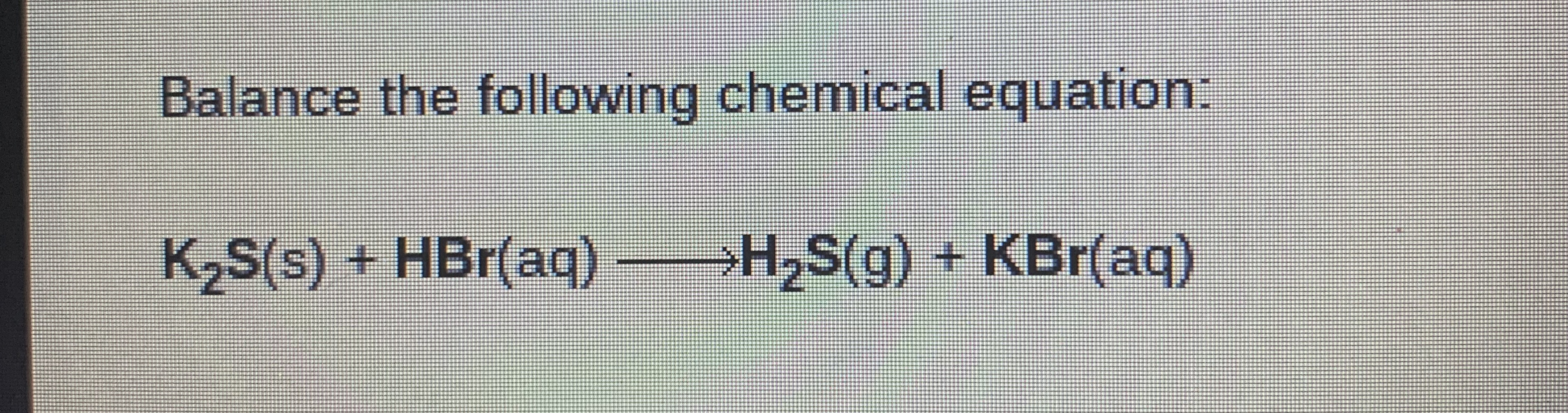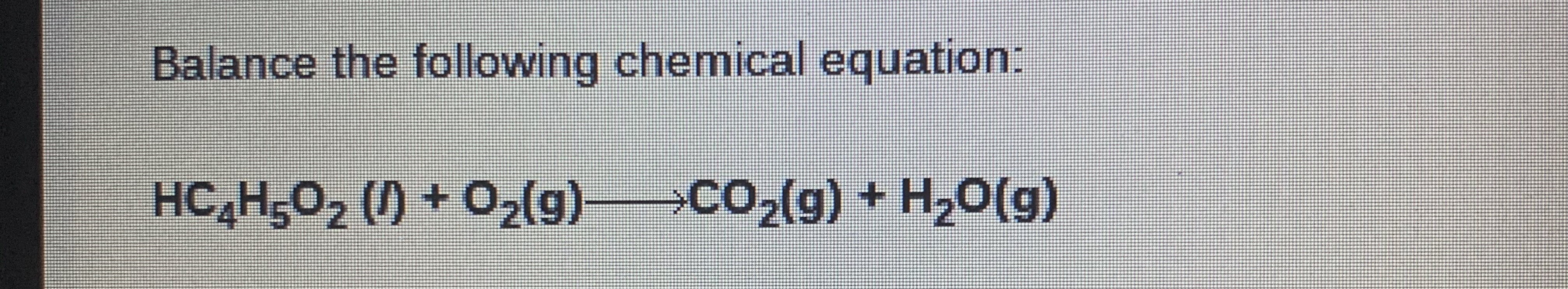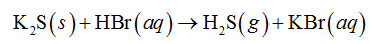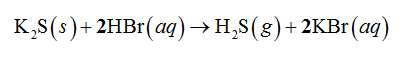# Balance the following chemical equation:K2S(s) + HBr(aq)>H2S(g) + KBr(aq) Balance the following chemical equation:HC H5O2 () + 02(g)>CO2(g) + H,0(g)

Question
1 viewshelp_outlineImage TranscriptioncloseBalance the following chemical equation: K2S(s) + HBr(aq) >H2S(g) + KBr(aq) fullscreenhelp_outlineImage TranscriptioncloseBalance the following chemical equation: HC H5O2 () + 02(g)>CO2(g) + H,0(g) fullscreen
check_circle

Concept Introductions:

A balanced chemical equation is that in which all the reactants and products are written with their stoichiometric coefficient.

Part I

Given chemical reaction:In the above reaction, two potassium is on the left side of reaction and two hydrogen is on the right side of reaction. The above chemical reaction is balanced as shown below....

### Want to see the full answer?

See Solution

#### Want to see this answer and more?

Solutions are written by subject experts who are available 24/7. Questions are typically answered within 1 hour.*

See Solution
*Response times may vary by subject and question.
Tagged in